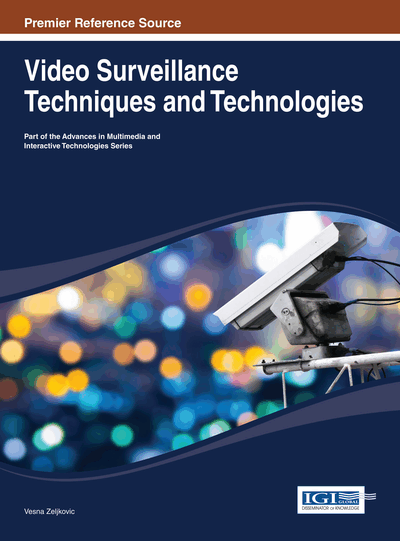# Classification of Building Images in Video Sequences

DOI: 10.4018/978-1-4666-4896-8.ch007
Available
\$37.50
No Current Special Offers

## Abstract

A technique for detection of building images in real-world video sequences is presented. The proposed technique uses information extracted from video features to improve precision in classification results. It combines fuzzy rule-based classification with a method for changing region detection in outdoor environments, which is invariant to extreme illumination changes and severe weather conditions. It has been tested on sequences under various lighting conditions. Satisfactory and promising results have been achieved.
Chapter Preview
Top

## 2. Edge-Based Building Image Classification

Edge-based building image classification can be found in (Iqbal, 1999), (Vailaya, 2001), (Wang, 2002).

Let x be an image or video frame, f = {f(1), . . ., f(n)} be feature sets where f is a function of the image x, Et = (e1t , . . ., e5t ) be a pattern extracted from a feature vector f(t), and Y = {B, NB} be a class set (B:Building, NB:Non Building). Edge-based building image classification uses a function:g(x): Et → Y (1) where Et is extracted from the description of x given by the MPEG-7 edge histogram descriptor.

This descriptor uses an 80-bin histogram to represent the local distribution of directional (vertical, horizontal, diagonal 45, and diagonal 135) and non- directional (isotropic) edges.

g(x) is approximated with a set R = {R1, . . ., RC } of if-then fuzzy rules which has the following structure:WRk: If eit is Aik then Y is yj(2) where w Rk is a weighted rule, w ∈ [0, 1] is the weight, eit a specific type of edge, and Ak is a linguistic label used to transform values from a continuous to a discrete domain.

The fuzzy model has five input variables because of the semantics of edge histogram descriptor which uses five types of edges. Besides, a group of three fuzzy sets is associated with each variable. Thus, rule base is a multi-input single-output space as is depicted in Figure 1.

Ri and Rj are if-then rules associated with classes NB and B, respectively. H, M, L stand for High, Low, Medium, and Low.

## Complete Chapter List

Search this Book:
Reset